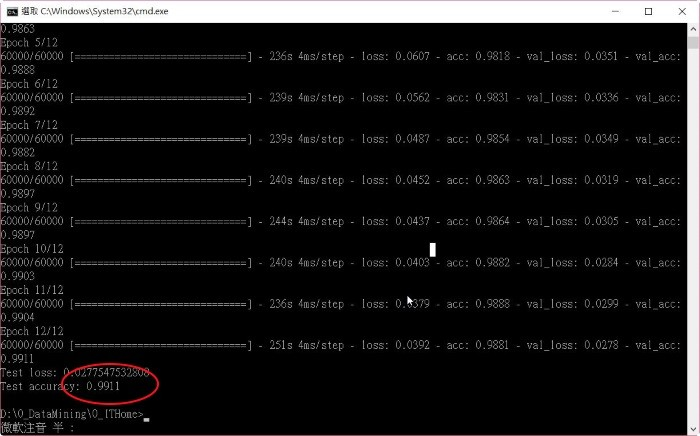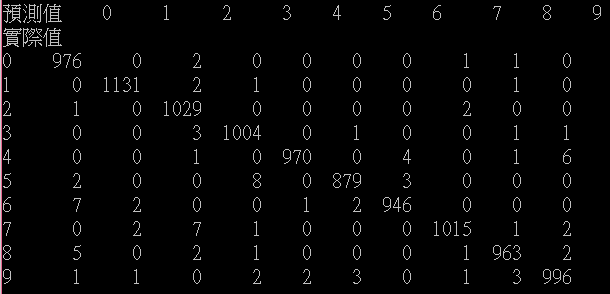2018 iT 邦幫忙鐵人賽DAY 7
1
AI & Machine Learning

範例程式

from __future__ import print_function
import keras
from keras.datasets import mnist
from keras.models import Sequential
from keras.layers import Dense, Dropout, Flatten
from keras.layers import Conv2D, MaxPooling2D
from keras import backend as K

# 定義梯度下降批量
batch_size = 128
# 定義分類數量
num_classes = 10
# 定義訓練週期
epochs = 12

# 定義圖像寬、高
img_rows, img_cols = 28, 28

# 載入 MNIST 訓練資料
(x_train, y_train), (x_test, y_test) = mnist.load_data()

# 保留原始資料，供 cross tab function 使用
y_test_org = y_test

# channels_first: 色彩通道(R/G/B)資料(深度)放在第2維度，第3、4維度放置寬與高
if K.image_data_format() == 'channels_first':
x_train = x_train.reshape(x_train.shape, 1, img_rows, img_cols)
x_test = x_test.reshape(x_test.shape, 1, img_rows, img_cols)
input_shape = (1, img_rows, img_cols)
else: # channels_last: 色彩通道(R/G/B)資料(深度)放在第4維度，第2、3維度放置寬與高
x_train = x_train.reshape(x_train.shape, img_rows, img_cols, 1)
x_test = x_test.reshape(x_test.shape, img_rows, img_cols, 1)
input_shape = (img_rows, img_cols, 1)

# 轉換色彩 0~255 資料為 0~1
x_train = x_train.astype('float32')
x_test = x_test.astype('float32')
x_train /= 255
x_test /= 255

# y 值轉成 one-hot encoding
y_train = keras.utils.to_categorical(y_train, num_classes)
y_test = keras.utils.to_categorical(y_test, num_classes)

# 建立簡單的線性執行的模型
model = Sequential()
# 建立卷積層，filter=32,即 output space 的深度, Kernal Size: 3x3, activation function 採用 relu
activation='relu',
input_shape=input_shape))
# 建立卷積層，filter=64,即 output size, Kernal Size: 3x3, activation function 採用 relu
model.add(Conv2D(64, (3, 3), activation='relu'))
# 建立池化層，池化大小=2x2，取最大值
# Dropout層隨機斷開輸入神經元，用於防止過度擬合，斷開比例:0.25
# Flatten層把多維的輸入一維化，常用在從卷積層到全連接層的過渡。
# 全連接層: 128個output
# Dropout層隨機斷開輸入神經元，用於防止過度擬合，斷開比例:0.5
# 使用 softmax activation function，將結果分類

# 編譯: 選擇損失函數、優化方法及成效衡量方式
model.compile(loss=keras.losses.categorical_crossentropy,
metrics=['accuracy'])

# 進行訓練, 訓練過程會存在 train_history 變數中
train_history = model.fit(x_train, y_train,
batch_size=batch_size,
epochs=epochs,
verbose=1,
validation_data=(x_test, y_test))

# 顯示損失函數、訓練成果(分數)
score = model.evaluate(x_test, y_test, verbose=0)
print('Test loss:', score)
print('Test accuracy:', score)

# 模型結構存檔
from keras.models import model_from_json
json_string = model.to_json()
with open("cnn.config", "w") as text_file:
text_file.write(json_string)

# 模型訓練結果存檔
model.save_weights("cnn.weight")# 計算『混淆矩陣』(Confusion Matrix)，顯示測試集分類的正確及錯認總和數
import pandas as pd
predictions = model.predict_classes(x_test)
pd.crosstab(y_test_org, predictions, rownames=['實際值'], colnames=['預測值'])程式說明

1. W: input 的寬度
2. F：濾波器數量
3. P：補零的策略，卷積層取週邊NxN的滑動視窗時，若超越邊界時，是否要放棄這個點、還是一律補零，若採後者，P就等於1，反之為0。
4. S：『滑動步長』(Stride)，指滑動視窗時，要一次滑動幾格。結語

1 則留言

0

score 也就是acc與自行計算混淆矩陣的準確率不相符，請問您知道有什麼原因可能造成這種狀況嗎?